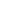# Free Class 8 Playing with Numbers Worksheets

Download free printable Playing with Numbers Worksheets to practice. With thousands of questions available, you can generate as many Playing with Numbers Worksheets as you want.

## Sample Class 8 Playing with Numbers Worksheet Questions

1.

If m7n5 is exactly divided by 3, then the least value of (x+y) is

1.

0

2.

2

3.

3

4.

4

2.

if 3x7 is exactly divisible by 3, then lease value of x is

1.

0

2.

1

3.

2

4.

4

3.

If 31z5 is a multiple of 3 then what cannot be the value of z?

1. 3 2. 6 3. 0 4. 2
4.

If 3A + 25 = B2,the value of B is

1. 3 2. 7 3. 6 4. 6
5.

If 3A + 25 = B2,the value of A is

1. 3 2. 7 3. 6 4. 9
6.

If 21y5 is a multiple of 9 then value of y is

1. 3 2. 2 3. 1 4. 4
7.
If the division 1234x 3 leaves a remainder of 1. What might be the least value of x?
1. 1 2. 2 3. 0 4. 5
8.
What is the difference between three digit largest and smallest number which is divisible by 3?
9.
What is the difference between two digit largest and smallest number which is divisible by 2?
10.
What should be subtracted from 35875 to make it exactly divisible by 11?
1. 1 2. 2 3. 3 4. 4

Worksheets by UrbanPro

Our worksheets are designed to help students explore various topics, practice skills and enrich their subject knowledge, to improve their academic performance. Designed by Experts who have extensive experience and expertise in teaching a subject, these worksheets will improve your child's problem-solving skills and subject knowledge in a fun and interactive manner.
Check out our free customized worksheets across school boards, grades, subjects and levels of subject knowledge. You can download, print and share these worksheets with anyone, anywhere, anytime!

Get a custom worksheet to practice!

Select your topic & see the magic.

subjectSelect Chapter(s)

Chapters & Subtopics